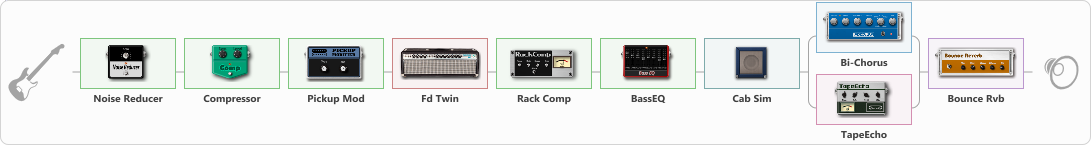# 90 Clean

Discussion in 'ToneLib-GFX presets' started by truss van halen, Jun 10, 2021.

1. ### truss van halenWell-Known Member

90 Clean

Preset name: 90''s truss Clean

Effects chain:Effect: "Noise Reducer" (Dynamics / Filter), active - "yes"
{
"Sens" = 83
"Mode" = Soft
}

Effect: "Compressor" (Dynamics / Filter), active - "yes"
{
"Sense" = 21
"Level" = 50
}

Effect: "Pickup Mod" (Dynamics / Filter), active - "yes"
{
"Mode" = Phase Out
"Tone" = 32
}

Effect: "Fd Twin" (Amp simulators), active - "yes"
{
"Gain" = 45
"Bass" = 74
"Middle" = 66
"Treble" = 36
"Presence" = 31
"Master" = 32
"Level (dB)" = 9
}

Effect: "Rack Comp" (Dynamics / Filter), active - "yes"
{
"Threshold (dB)" = 6
"Ratio" = 1
"Attack" = Slow
"Release (ms)" = 70
"Knee" = 13
"Level (dB)" = 0
}

Effect: "BassEQ" (Dynamics / Filter), active - "yes"
{
"50 Hz" = 4
"120 Hz" = 0
"400 Hz" = -1
"500 Hz" = -2
"800 Hz" = -4
"4.5 kHz" = -2
"10 kHz" = 2
"Level (dB)" = 0
}

Effect: "Cab Sim" (Cabinets), active - "yes"
{
"Model" = 2x12" Silver Bell
"Level (dB)" = 6
}

Effect: "Splitter" (Dynamics / Filter)
{
"A-Bypass" = Off
"A-Pan" = 0
"A-Level" = 55
"B-Bypass" = Off
"B-Pan" = 0
"B-Level" = 55

'A' branch:
{

Effect: "Bi-Chorus" (Modulation / Sfx), active - "yes"
{
"Speed A" = 3.2
"Speed B" = 4.0
"Depth" = 43
"Reso" = 0
"Mode" = Parallel
"Mix" = 67
}
}
'B' branch:
{

Effect: "TapeEcho" (Delay), active - "yes"
{
"Time" = 498
"Feedback" = 50
"Tone" = 65
"LoDamp" = 19
"Mix" = 65
}
}
}

Effect: "Bounce Rvb" (Reverberation), active - "yes"
{
"Time" = 8.6
"PreDelay" = 71
"LoDamp" = 24
"HiDamp" = 42
"Mix" = 67
}

Note: You will need to download and install the ToneLib-GFX software to use the preset.

#### Attached Files:

• ###### 90_Clean.tlgfx
File size:
1,011 bytes
Views:
359
renayuk, KAZGROOVE and nikolay like this.